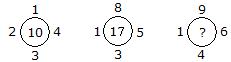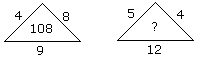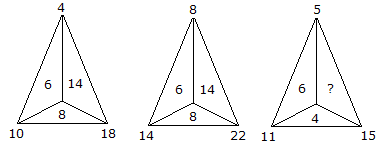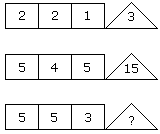# Verbal Reasoning - Character Puzzles

### Exercise :: Character Puzzles - Character Puzzles 1

51.

Which one will replace the question mark ?A. 18 B. 20 C. 21 D. 19

Explanation:

1 + 2 + 3 + 4 = 10

and 1 + 3 + 5 + 8 = 17

Similarly, 1 + 4 + 6 + 9 = 20.

52.

Which one will replace the question mark ?A. 80 B. 114 C. 108 D. None of these

Explanation:

(4 + 8) x 9 = 108

(5 + 4) x 12 = 108.

53.

Which one will replace the question mark ?A. 8 B. 14 C. 10 D. 6

Explanation:

For first triangle,

10 - 4 = 6

18 - 10 = 8

18 - 4 = 14

For second triangle,

14 - 8 = 6

22 - 14 = 8

22 - 8 = 14

For third triangle,

11 - 5 = 6

15 - 11 = 4

15 - 5 = 10.

54.

Which one will replace the question mark ?A. 11 B. 19 C. 15 D. 22

Explanation:

(2 x 2 - 1) = 3

and (5 x 4 - 5) = 15

(5 x 5 - 3) = 22.